Courses

# Test: Partnership 5

## 20 Questions MCQ Test Quantitative Techniques for CLAT | Test: Partnership 5

Description
This mock test of Test: Partnership 5 for Quant helps you for every Quant entrance exam. This contains 20 Multiple Choice Questions for Quant Test: Partnership 5 (mcq) to study with solutions a complete question bank. The solved questions answers in this Test: Partnership 5 quiz give you a good mix of easy questions and tough questions. Quant students definitely take this Test: Partnership 5 exercise for a better result in the exam. You can find other Test: Partnership 5 extra questions, long questions & short questions for Quant on EduRev as well by searching above.
QUESTION: 1

### A, B, C started a business with their investments in the ratio 1 : 3 : 5. After 4 months, A invested the same amount as before and B as well as C withdrew half of their investments. The ratio of their profits at the end of the year is ?

Solution: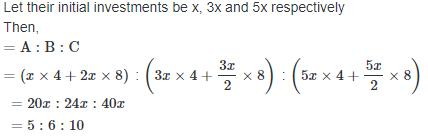QUESTION: 2

### In a partnership, A invests 1/6 of the capital 1/6 for of the time, B invests 1/3 of the capital for 1/3 of the time and C, the rest of the capital for the whole time. Out of a profit of Rs. 4600, B's share is ?

Solution: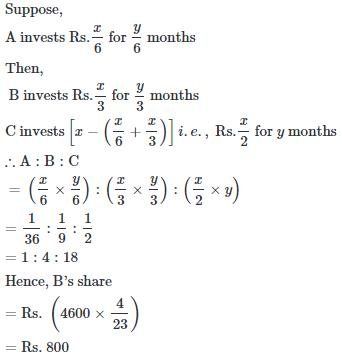QUESTION: 3

### A starts business with a capital of Rs. 14000. Five months later B joins and further two months later C joins them. If the profit sharing ratio in the end of year is 4 : 3 : 2, then the money invested by C was ?

Solution: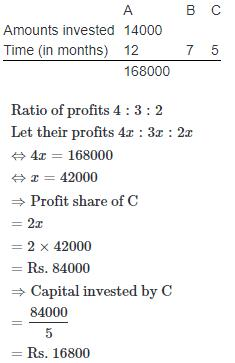QUESTION: 4

A, B and C become partners in a business. A contributes 1/3 rd of the capital for 1/4 th of the time. B contributes 1/5 th of the capital for 1616 th of the time and C the rest of the capital for the whole time. If the profit is Rs. 1820, then the A's share in profit is ?

Solution: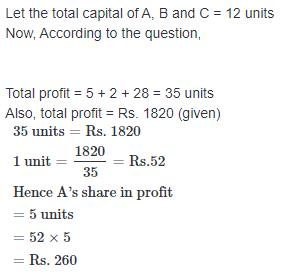QUESTION: 5

A, B and C entered into a partnership. A invested Rs. 2560 and B invested Rs. 2000. At the end of the year, they gained Rs. 1105, out of which A got Rs. 320. C's capital was ?

Solution: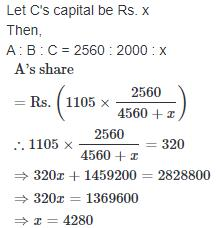QUESTION: 6

A, B and C enter into a partnership. A contributes one-third of the capital while B contributes as much as A and C together contribute. If the profit at the end of the year amounts to Rs. 900, what would C receive ?

Solution: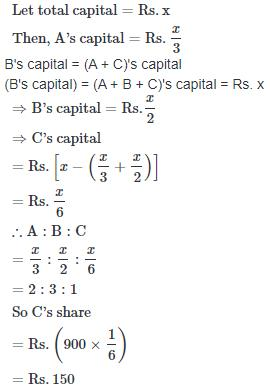QUESTION: 7

A and B started a business jointly. A's investment was thrice the investment of B and the period of his investment was two times the period of investment of B. If B received Rs. 4000 as profit, then their total profit is ?

Solution: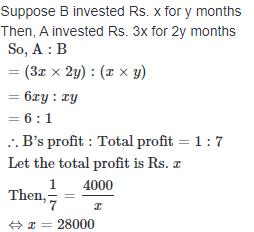QUESTION: 8

A began a business with Rs. 85000, he was joined afterwards by B with Rs. 42500. For how much period does B join, if the profits at the end of the year are divided in the ratio of 3 : 1 ?

Solution: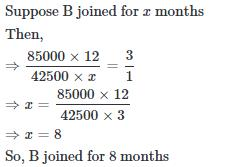QUESTION: 9

A and B share profits and losses in a firm in the ratio of 3 : 2. And C entered in the firm as a new partner; his profit sharing ratio is 1/4. If C has taken his share of profit from A and B in equal ratio, then the new profit shearing ratio will be ?

Solution: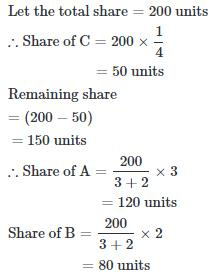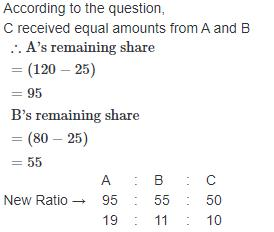QUESTION: 10

A, B and C share the profit in the ratio of 2 : 3 : 7. If the average gain is Rs. 8000, then B's share is ?

Solution: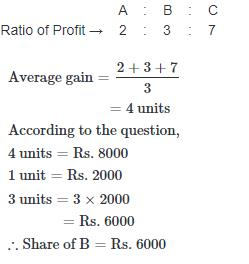QUESTION: 11

A and B enter into a partnership with Rs. 50000 and Rs. 60000 respectively. C joins them after x months, contributing Rs. 70000 and B leaves x months before the end of the year. If they share the profit in the ratio of 20 : 18 : 21, then the value of x is = ?

Solution: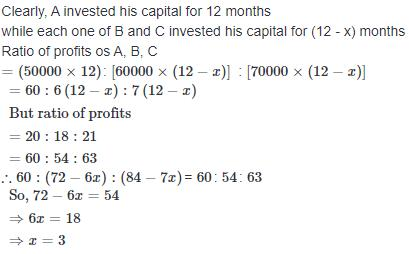QUESTION: 12

Two friends P and Q started a business investing in the ratio 5 : 6. R joined them after six months investing an amount equal to that of Q's. At the end of the year, 20% profit was earned which was equal to Rs. 98000. What was the amount invested by R?

Solution: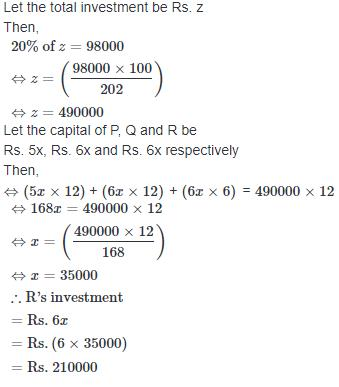QUESTION: 13

A, B and C invested their capitals in the ratio 3 : 4 : 6. However their share of profit are equal. The duration of their investments must be in the ratio ?

Solution: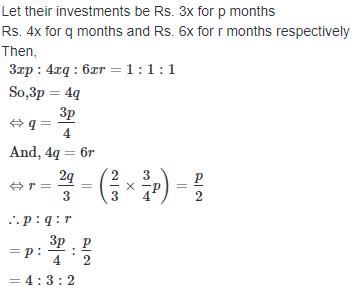QUESTION: 14

Swati and Rajni enter into a partnership with their capitals in the ratio 5 : 6. At the end of 7 months Swati withdraws her capital. If they receive the profit in the ratio of 5 : 9, find how long was Rajni's capital used ?

Solution: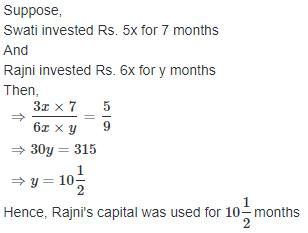QUESTION: 15

X and Y are partners in a business. X contributed 1/3 of the capital for 9 months and Y received 2/5 of the profit. For how long was Y's money used in the business ?

Solution: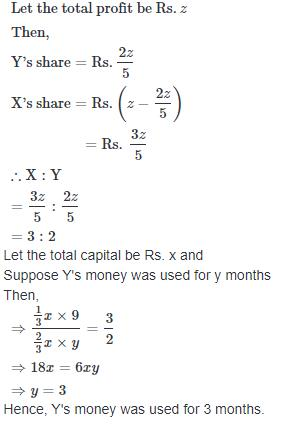QUESTION: 16

M, P and Q together started a business. M invested Rs. 6500 for 6 months, P invested Rs. 8400 for 5 months and Q invested Rs. 10000 for 3 months. M is working member for which he gets 5% of total profit extra. If the total gain is Rs. 7400, then Q's share is ?

Solution: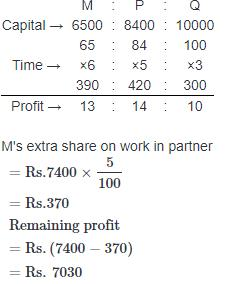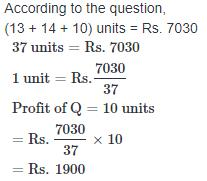QUESTION: 17

A started a business by investing Rs. 50000. After 6 months B joined her by investing Rs. 75000. After its 6 months C joined with Rs. 125000. What is the ratio of profit share after 2 year among A, B and C ?

Solution: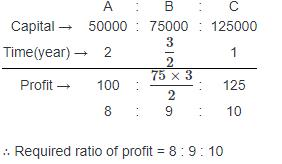QUESTION: 18

A and B started a business with initial investments in the respective ratio of 18 : 7. After 4 months from the start of the business, A invested Rs. 2000 more and B invested Rs. 7000 more. At the end of one year, if the profit was distributed among them in the ratio of 2 : 1 respectively, what was the total initial investment with which A and B started the business ?

Solution:

Let the initial investment of A and B is 18x and 7x
After 4 months from the start of business,
A invest Rs. 2000 more for each eight months.
Then total investment of A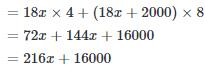After 4 months, from the start of business,
B invest Rs. 7000 more for each eight months.
Total investment by B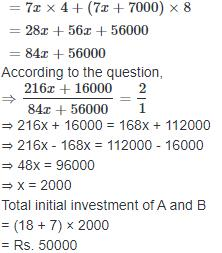QUESTION: 19

Anil, Kamal and Vini invested Rs. 8000, Rs. 4000 and Rs. 8000 respectively in a business. Anil left after 6 months. If after 8 months, there was a gain of Rs. 4005, then what will be the share of Kamal ?

Solution:

Ratio of profit of Anil : Kamal : Vini
(8000 × 6) : (4000 × 8) : (8000 × 8)
= 48000 : 32000 : 64000
= 48 : 32 : 64
= 3 : 2 : 4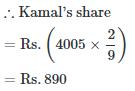QUESTION: 20

A starts a business by investing Rs. 28000. After 2 months, B joins with Rs. 20000 and after another 2 months C joins with Rs. 18000. At the end of 10 months from the start of the business, if B withdraws Rs. 2000 and C withdraws Rs. 2000, in what ratio should the profit be distributed among A, B and C at the end of the year ?

Solution:

A invests money for 12 months
B invests money for 10 months
C invests money for 8 months
Ratio of profit of A to B to C
= 28000 × 12:20000 × 8 + 18000 × 2 : 18000 × 6 + 16000 × 2
= 28 × 12 × 1000 : (160 + 36) × 1000 : (108 + 32) × 1000
= 28 × 12 : 160 + 36 : 108 + 32
= 336 : 196 : 140
= 12 : 7 : 5